# Displacement¶

Use the Displacement material to displace the surface of objects. The surface is moved along its normal according to Amount.

Textures are usually a source of displacement, but you can also use sub-shaders such as weavings, stripes, Library/Attributes/Cloud or any custom sub-shader.

### Amount

The Amount attribute is the displace amount you want to control with a texture or a procedural.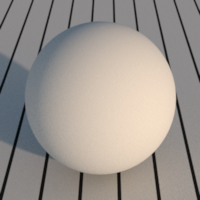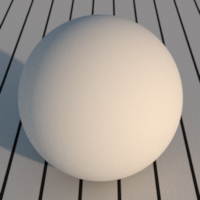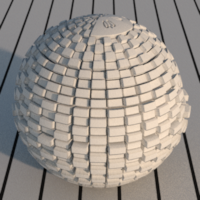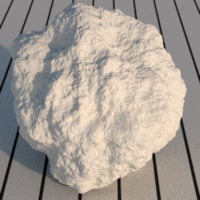The Displacement > Amount set to 0.1, 0.5, driven by a Library/Attributes/MaskTexture and by a Library/Attributes/Cloud.

### Normalization, Offset and Multiplier

The Normalization attribute describes how the displacement is computated:

• Normalize : The displacement distance is equal to clamp((Amount-Offset)*Multiplier,-1,+1)*DisplacementAmount, that is the input Amount is offseted and scaled with Offset and Multiplier, then clamped to -1,1 and eventually scaled by the Attributes > Displacement > Displacement Amount attribute. Use this mode if your displacement map is a classic greyscale map.
• Raw : The displacement distance is equal to Amount. Use this mode if your displacement map is an absolute float map.
• Affine : The displacement distance is equal to (Amount-Offset)*Multiplier. Use this mode if your displacement map is an absolute float map, but you need to tweak the resulting value.
Raw and Affine modes require you to correctly set the Attributes > Displacement > Displacement Amount attribute to a value larger than the maximum displacement, in order to avoid holes in the renders.

### Compute Normals

If checked, recompute the normals after the displacement, else keep the original normals. Turn this attribute off if you also use a normal or a bump texture which already contains the displacement normals.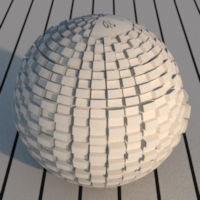The Displacement > Compute Normals set to On and Off.